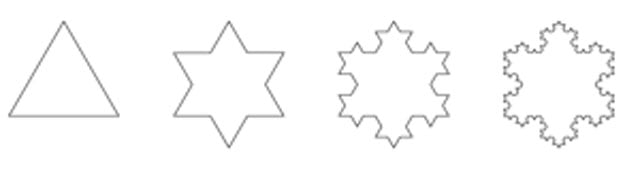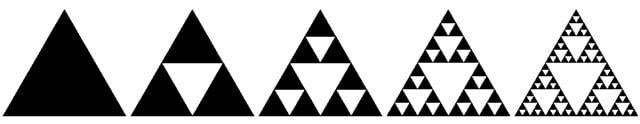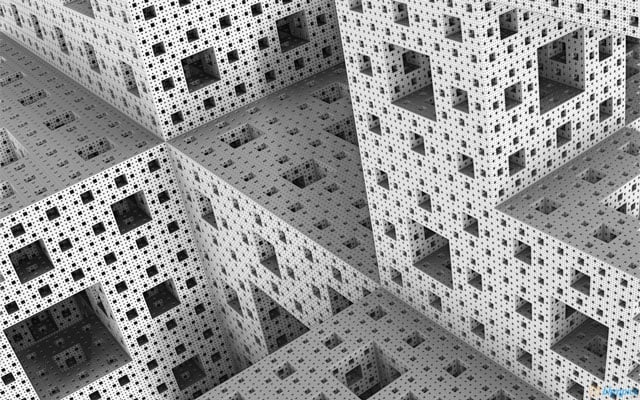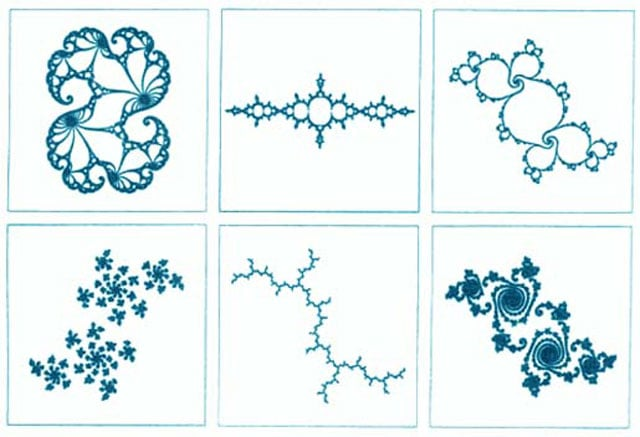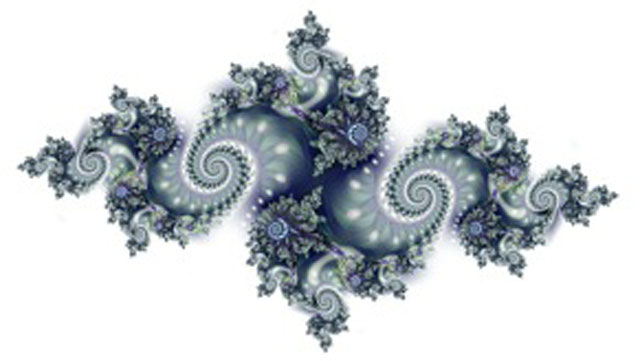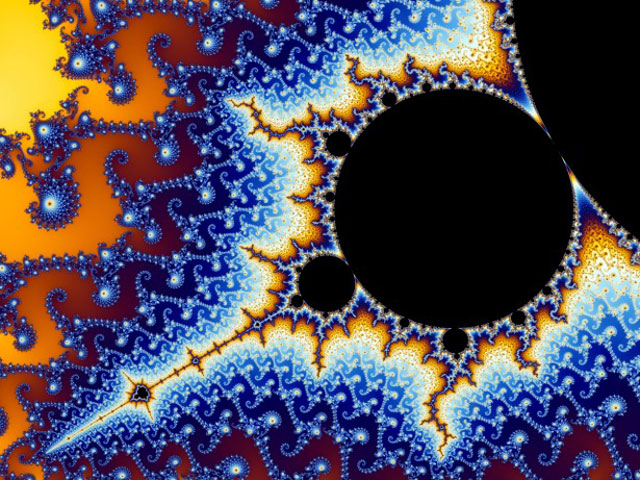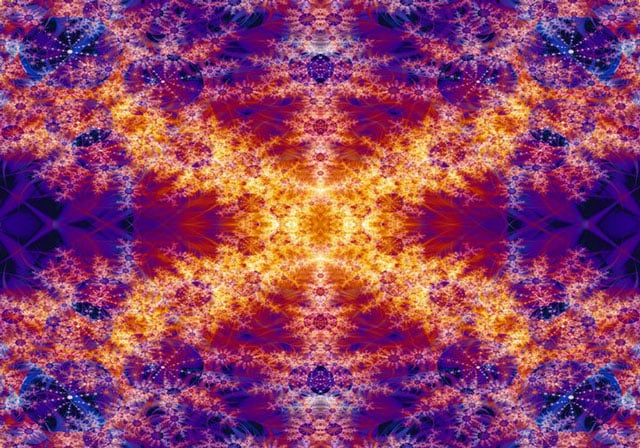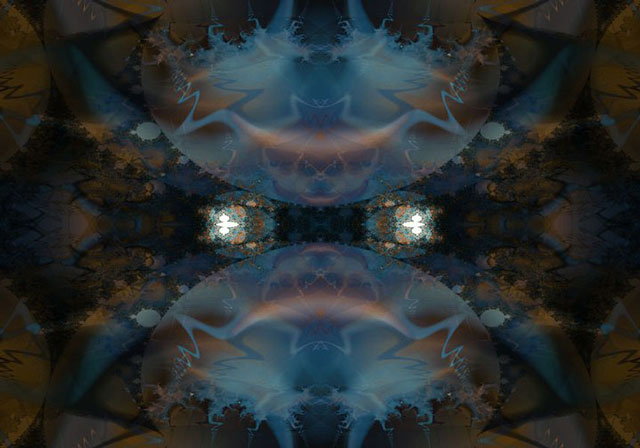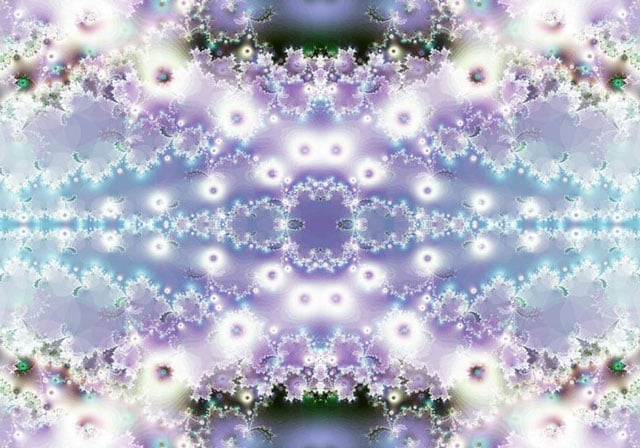Maths, beauty and art are all related! Would you believe that all these different images are called fractals, and they are created through different mathematical equations?! While many people have difficulty understanding or liking maths, those who give fractals a chance are rarely disappointed.

Our senior developer Dan gave us this great website for explaining how fractals work. If you have Google Chrome, give it a try, you won't regret it!

With the growth of html5 technologies such as WebGL and Canvas, dynamically scalable fractals are now possible to be created in the online space. A good example of this is here, where the Google Maps API zooming and panning functionality is utilised.

According to another fascinating fractal website, "what differentiates fractal art from other forms of digital art is that mathematics plays a much greater role in producing fractal images than it does in other forms. The other forms are not devoid of math--far from it, most things connected with computers use mathematics at some level--but mathematics occupies a special place for fractals, because the shapes that are so prevalent in fractal art are precisely defined by mathematics."

Regardless of how much maths freak you out, consider giving fractals and the maths behind them a chance. You might even think about creating something mathematically magnificent!

The sources from these images are listed in the order they appear below.The last three fractals seen here were created by Dan through Fractal Explorer!